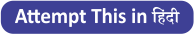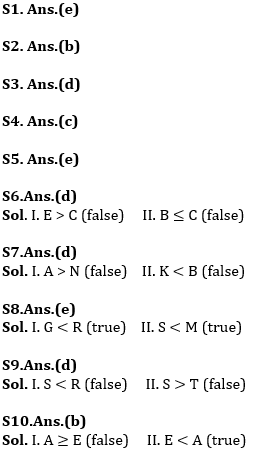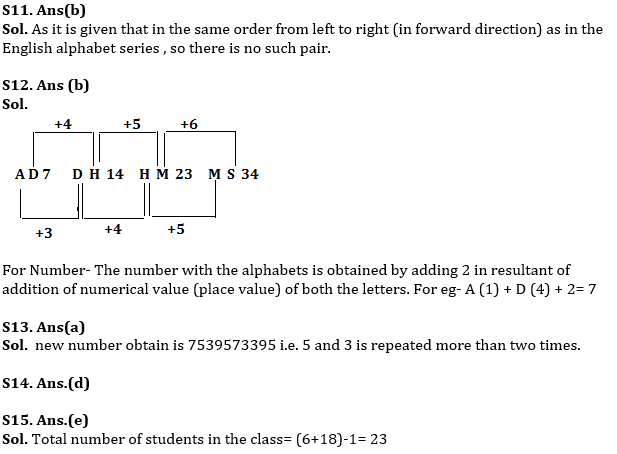Latest Banking jobs   »   Revision Test Basic Reasoning Quiz for...

# Revision Test Basic Reasoning Quiz for All Banking Exams- 05th May

Directions (1-5): These questions are based on the following arrangement. Study it carefully and answer the questions below it.

B 7 E H \$ 3 L % 8 I F M 4 1 A R 6 # ↑ 9 D 5 K U 2 T

Q1. Which element is exactly midway between seventh from the left end and sixth from the right end?
(a) A
(b) R
(c) 4
(d) 6
(e) 1

Q2. If from the above arrangement all the numbers are deleted, which one will be fourth to the right of tenth from the right end?
(a) ↑
(b) #
(c) R
(d) D
(e) None of these

Q3. How many such vowels are there in the above arrangement, each of which is immediately preceded by a consonant and also immediately followed by a number?
(a) None
(b) Three
(c) Two
(d) One
(e) More than three

Q4. How many such symbols are there in the given arrangement, each of which is immediately followed by a number, but not immediately preceded by another symbol?
(a) None
(b) One
(c) Two
(d) Three
(e) More than three

Q5. Four of the following five are alike in a certain way based on their positions in the given arrangement and so form a group. Which is the one that does not belong to the group?
(a) EBH
(b) 8LI
(c) 2KT
(d) #R↑
(e) 41F

Directions (6-10): In these questions, the relationship between different elements is shown in the statements. The statements are followed by two conclusions. Give answer
(a) if only conclusion I is true
(b) if only conclusion II is true
(c) if either conclusion I or II is true
(d) if neither conclusion I nor II is true
(e) if both conclusions I and II are true.

Q6. Statements: G ≤ C, B > G, E > A ≥ K, B< K
Conclusions:
I. E > C II. B ≤ C

Q7. Statements: A ≥ K, K< M > N, B ≥ N
Conclusions:
I. A > N II. K < B

Q8. Statements: G > S, M ≥ N > G, R ≥ M
Conclusions:
I. G < R II. S < M

Q9. Statements: N ≥ M < S, R > T < J, N > R
Conclusions:
I. S < R II. S > T

Q10. Statements: B ≤ A < D, C ≥ E, B > C
Conclusions:
I. A ≥ E II. E < A

Q11. How many such pairs of letters are there in the word ‘PUBLICATION’ each of which has as many letters between them in the word as in the English alphabet and also in the same order from left to right (in forward direction) as in the English alphabet series ?
(a) One
(b) None
(c) Two
(d) Three
(e) More than three

Q12. If in a certain pattern, there is a series, find the next elements which will come in the place of ? according to that pattern of the series?
(a) MS32
(b) MS34
(c) HS34
(d) MR31
(e) None of these

Q13. If 2 is subtracted from each odd digit in the number 9758462587 and 1 is added to each even digit in number then how many digits of the following number is repeated more than three times in the new number so obtained?
(a) none
(b) one
(c) two
(d) three
(e) more than three

Q14. In a row Shivani is 20th from the left and Monica is 20th from the right. If they interchange their positions then Shivani become 20th from the right end. Then find How many persons are in the row?
(a) 40
(b) 39
(c) 41
(d) CND
(e) None of these

Q15. Dinesh rank is 6th from the top and eighteenth from the bottom in the class. How many students are there in the class?
(a) 29
(b) 28
(c) 27
(d) 25
(e) None of these

Practice More Questions of Reasoning for Competitive Exams:

Solutions#### Congratulations!Incorrect details? Fill the form again here

•Important Current Affairs Quiz for Bank ...
•Important Current Affairs Quiz for Bank ...
•Reasoning Ability Quiz For IBPS RRB PO C...
•Quantitative Aptitude Quiz For IBPS RRB ...
•Important Current Affairs Quiz for Bank ...
•Reasoning Ability Quiz For IBPS Clerk Pr...